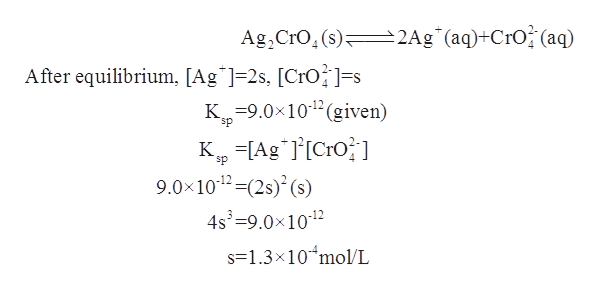# The Ksp for silver chromate (Ag2CrO4) is 9.0 ✕ 10−12. Calculate the solubility of silver chromate in each of the following.(a)    watermol/L(b)    0.25 M AgNO3mol/L(c)    0.38 M Na2CrO4mol/L

Question
26 views

The Ksp for silver chromate (Ag2CrO4) is 9.0 ✕ 10−12. Calculate the solubility of silver chromate in each of the following.

(a)    water
mol/L

(b)    0.25 M AgNO3
mol/L

(c)    0.38 M Na2CrO4
mol/L
check_circle

star
star
star
star
star
1 Rating
Step 1

The term solubility product constant can be defined as the equilibrium constant for the equilibrium present between a solid and its respective ions in the solution. The value of solubility product constant is used to indicate the degree up to which a given compound can dissociate in water.

Step 2

Let ‘s’ be the solubility of the ions present in Ag2CrO4 . Therefore, in water, its ions are dissociated as follows:help_outlineImage Transcriptionclose2Ag*(aq)+CrO? (aq) Ag, Cro, (s) After equilibrium, [Ag*]=2s, [CrO?]=s K=9.0x10" (given) sp K, =[Ag*l[Cro} ] 9.0x1012 =(2s) (s) 4s =9.0x1012 sp s=1.3×10*mol/L fullscreen
Step 3

The solubility of silver chromate in water is fo...

### Want to see the full answer?

See Solution

#### Want to see this answer and more?

Solutions are written by subject experts who are available 24/7. Questions are typically answered within 1 hour.*

See Solution
*Response times may vary by subject and question.
Tagged in

### Physical Chemistry